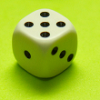#### You may also likeFour fair dice are marked differently on their six faces. Choose first ANY one of them. I can always choose another that will give me a better chance of winning. Investigate.### How Many Dice?

A standard die has the numbers 1, 2 and 3 are opposite 6, 5 and 4 respectively so that opposite faces add to 7? If you make standard dice by writing 1, 2, 3, 4, 5, 6 on blank cubes you will find there are 2 and only 2 different standard dice. Can you prove this ?### Dicing with Numbers

In how many ways can you arrange three dice side by side on a surface so that the sum of the numbers on each of the four faces (top, bottom, front and back) is equal?

# Troublesome Dice

##### Age 11 to 14 Challenge Level:

Mustafa from Wilson's School sent us this excellent and comprehensive solution.

Michael, also from Wilson's, came to the same conclusion about the possible results when the dice lands on an edge:

If two sides were showing, the following scores could be possible:

 + 1 2 3 4 5 6 1 - 3 4 5 6 - 2 - - 5 6 - 8 3 - - - - 8 9 4 - - - - 9 10 5 - - - - - 11 6 - - - - - -

The reason there are no numbers down the left is because 1+2 is the same as 2+1, etc.

There is one 3, one 4, two 5's, two 6's, NO 7's (since the numbers adding up to 7 are on opposite sides of the dice), two 8's, two 9's, one 10 and one 11.

Altogether there are 12 possibilities; 12 is divisible by the 3 family members and can therefore be split equally and fairly between them.

Matthew from The King's School in Grantham followed this up by showing how the numbers could be shared out:

They could be allocated like the following:

'Person A': 3, 4, 10, 11. Total of 4 chances.
'Person B': 5, 6. Total of 4 chances.
'Person C': 8, 9. Total of 4 chances.

If you have 2 people at the table, they could be split like the following:

'Person A': 3, 4, 5, 6. Total of 6 chances.
'Person B': 8, 9, 10, 11. Total of 6 chances.

If you have 4 people at the table, they could be allocated like the following: 'Person A': 3, 6. Total of 3 chances.
'Person B': 4, 5. Total of 3 chances.
'Person C': 8, 10. Total of 3 chances.
'Person D' : 9, 11. Total of 3 chances.

IT IS IMPOSSIBLE TO HAVE 5 PEOPLE AT THE TABLE, AND SHARE THE TOTALS OUT FAIRLY.

If you have 6 people at the table, the totals could be shared out fairly.

Rian and Ben from Waverley School agreed:

Sum of scores on the edge:

Chances of getting
3 = $\frac{1}{12}$
4 = $\frac{1}{12}$
5 = $\frac{2}{12}$
6 = $\frac{2}{12}$
8 = $\frac{2}{12}$
9 = $\frac{2}{12}$
10 = $\frac{1}{12}$
11 = $\frac{1}{12}$

You can split them fairly:
if person 1 gets a score of 3, 4, 10 or 11 the chances of winning are $\frac{4}{12}$
and then person 2 gets a score of 5 or 8 the chances of winning is $\frac{4}{12}$
and then person 3 gets 6 or 9 and the chances of winning are $\frac{4}{12}$.

Sum of scores at the corner:

For this situation, you cannot split them fairly because each score is unique so the probability of getting each number is $\frac{1}{8}$.

There are 8 numbers and 3 people so you can't split it fairly but you can split it between 2, 4 and 8 people.

Irene and Remminbi from Dulwich College in Beijing and Callum from Cholsey Primary School also sent us partial solutions to this problem.
Well done to you all.